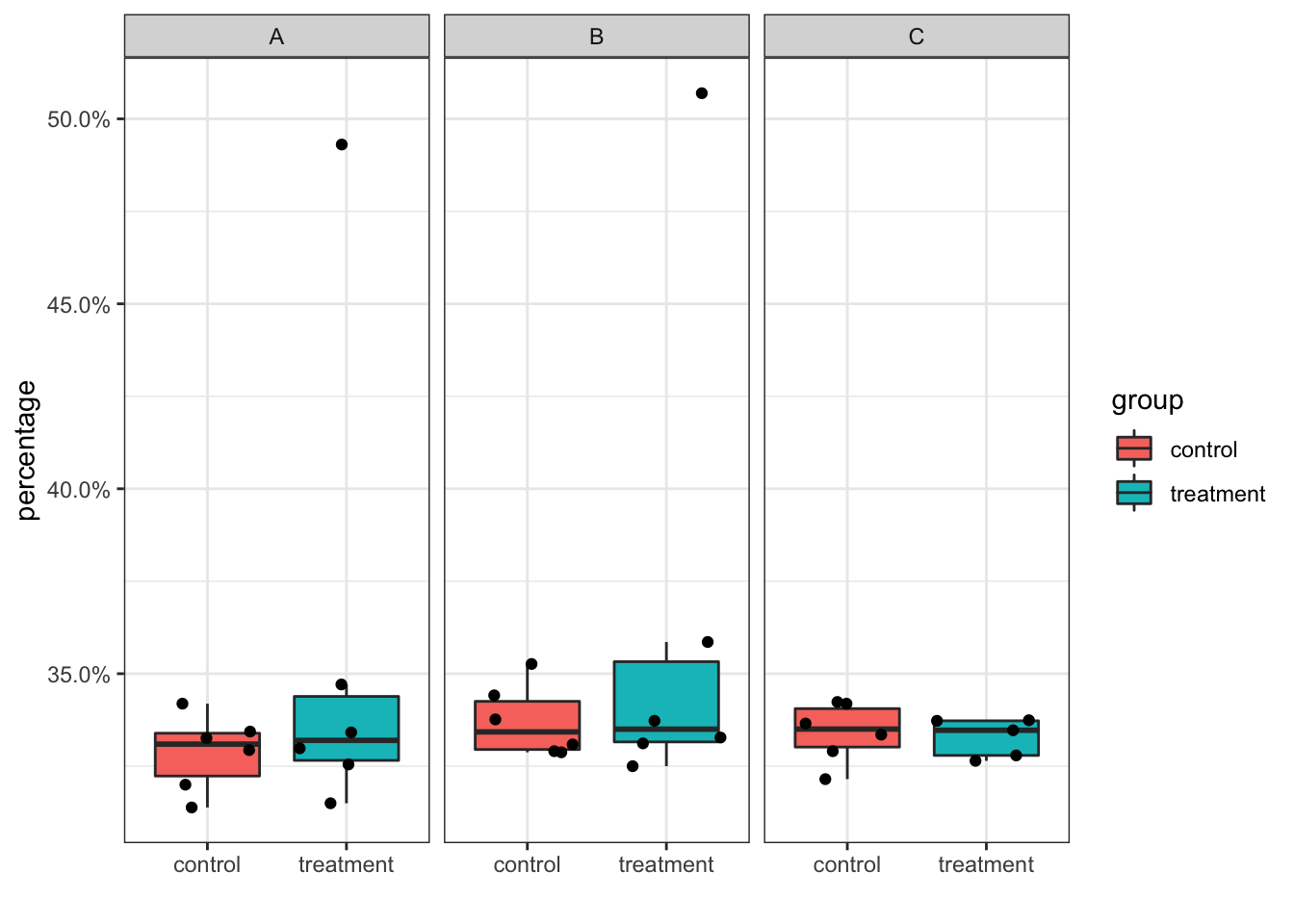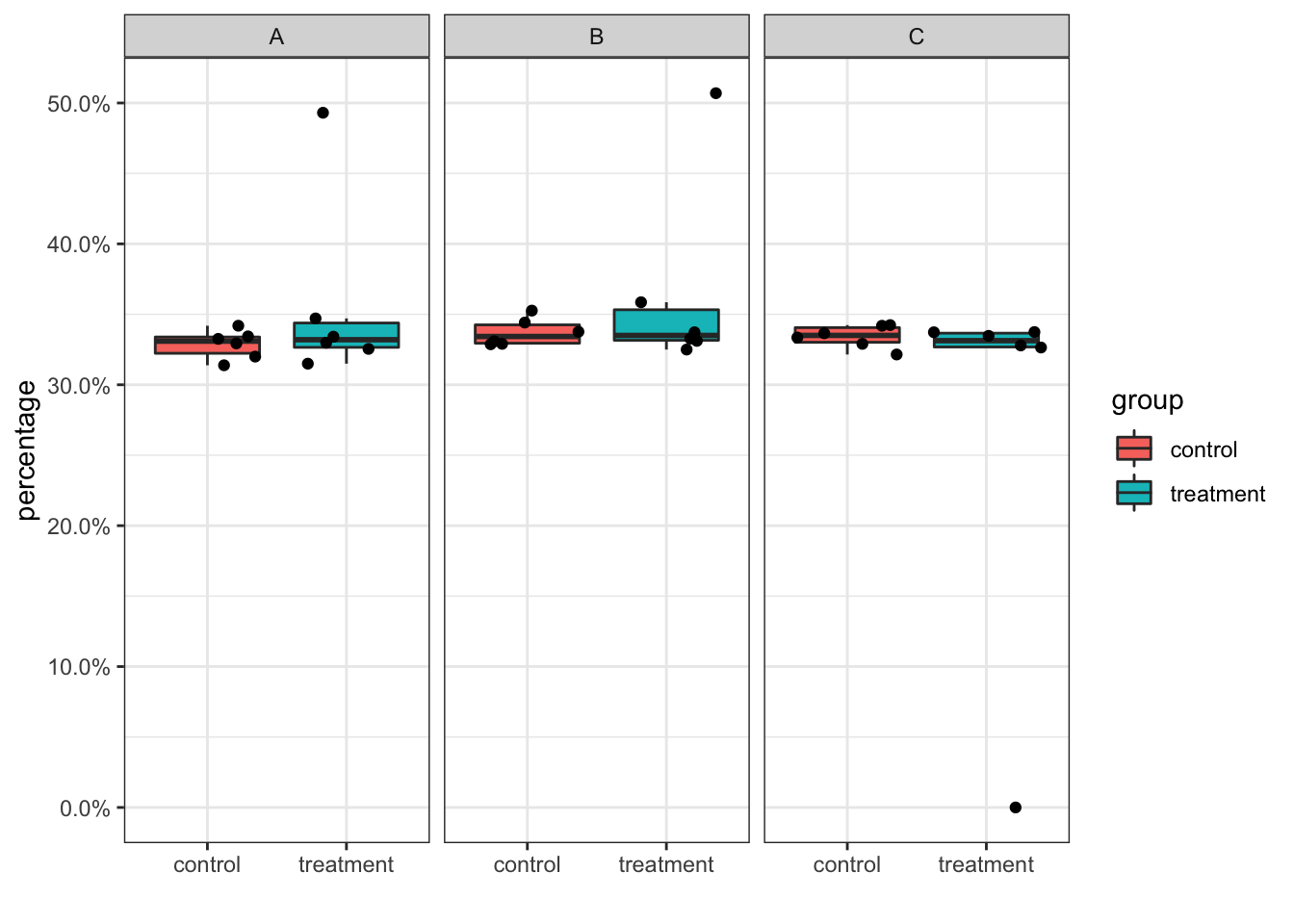# dplyr::count misses factor levels: a case in comparing scRNAseq cell type abundance

It is very common to see in the scRNAseq papers that the authors compare cell type abundance across groups (e.g., treatment vs control, responder vs non-responder).

Let’s create some dummy data.

library(tidyverse)
set.seed(23)

# we have 6 treatment samples and 6 control samples, 3 clusters A,B,C
# but in the treatment samples, cluster C is absent (0 cells) in sample7
sample_id<- c(paste0("sample", 1:6, "_control", rep(c("_A","_B","_C"),each = 6)), paste0("sample", 8:12, "_treatment", rep(c("_A","_B", "_C"), each = 5)))

sample_id<- c(sample_id, "sample7_treatment_A", "sample7_treatment_B")
cell_id<- paste0("cell", 1:20000)

cell_df<- tibble::tibble(sample_id = sample(sample_id, size = length(cell_id), replace = TRUE),
cell_id = cell_id) %>%
tidyr::separate(sample_id, into = c("sample_id", "group", "cluster_id"), sep= "_")

cell_num<- cell_df %>%
group_by(group, cluster_id, sample_id)%>%
summarize(n=n())

cell_num
## # A tibble: 35 x 4
## # Groups:   group, cluster_id 
##    group   cluster_id sample_id     n
##    <chr>   <chr>      <chr>     <int>
##  1 control A          sample1     551
##  2 control A          sample2     546
##  3 control A          sample3     544
##  4 control A          sample4     585
##  5 control A          sample5     588
##  6 control A          sample6     542
##  7 control B          sample1     550
##  8 control B          sample2     562
##  9 control B          sample3     574
## 10 control B          sample4     563
## # … with 25 more rows
total_cells<- cell_df %>%
group_by(sample_id) %>%
summarise(total = n())

total_cells
## # A tibble: 12 x 2
##    sample_id total
##    <chr>     <int>
##  1 sample1    1673
##  2 sample10   1713
##  3 sample11   1691
##  4 sample12   1696
##  5 sample2    1633
##  6 sample3    1700
##  7 sample4    1711
##  8 sample5    1768
##  9 sample6    1727
## 10 sample7    1225
## 11 sample8    1720
## 12 sample9    1743

join the two dataframe to get percentage of cells per cluster per sample

cell_percentage<- left_join(cell_num, total_cells) %>%
mutate(percentage = n/total)
## Joining, by = "sample_id"
cell_percentage
## # A tibble: 35 x 6
## # Groups:   group, cluster_id 
##    group   cluster_id sample_id     n total percentage
##    <chr>   <chr>      <chr>     <int> <int>      <dbl>
##  1 control A          sample1     551  1673      0.329
##  2 control A          sample2     546  1633      0.334
##  3 control A          sample3     544  1700      0.32
##  4 control A          sample4     585  1711      0.342
##  5 control A          sample5     588  1768      0.333
##  6 control A          sample6     542  1727      0.314
##  7 control B          sample1     550  1673      0.329
##  8 control B          sample2     562  1633      0.344
##  9 control B          sample3     574  1700      0.338
## 10 control B          sample4     563  1711      0.329
## # … with 25 more rows

Let’s plot a boxplot

cell_percentage %>%
ggplot(aes(x = group, y = percentage)) +
geom_boxplot(outlier.shape = NA, aes(fill = group)) +
geom_jitter() +
scale_y_continuous(labels = scales::percent) +
facet_wrap(~cluster_id) +
theme_bw()+
xlab("")YES, if you are careful enough, you will find the treatment group in cluster C only contains 5 points. Because if a cluster is completely missing for a sample, there will not be any cells in the original cell_df. However, the percentage should be 0% for that data point and you should show it in the boxplot as the jitter point. Otherwise, the result can be misleading. You can spot on such mistakes when you plot out the points on top of the boxplot.

### How to fix it

The trick is to make the factor contains all the levels of all the combinations. When use group_by or count, add .drop =FALSE.

sample_id<- paste0("sample", 1:12)
cluster_id<- c("A","B","C")

factor_levels<- tidyr::expand_grid(sample_id, cluster_id) %>%
mutate(group = c(rep("control", 18), rep("treatment", 18))) %>%
mutate(sample_id = paste(sample_id, cluster_id, group, sep="_"))

cell_num2<- cell_df %>%
mutate(sample_id = paste(sample_id, cluster_id, group, sep="_")) %>%
mutate(sample_id = factor(sample_id, levels = factor_levels\$sample_id)) %>%
group_by(sample_id, .drop=FALSE) %>%
summarise(n=n()) %>%
tidyr::separate(sample_id, c("sample_id", "cluster_id", "group"))

## the 0 correctly showed up
cell_num2 %>%
filter(sample_id == "sample7")
## # A tibble: 3 x 4
##   sample_id cluster_id group         n
##   <chr>     <chr>      <chr>     <int>
## 1 sample7   A          treatment   604
## 2 sample7   B          treatment   621
## 3 sample7   C          treatment     0

Let’s replot the boxplot and see the difference:

cell_percentage<- left_join(cell_num2, total_cells) %>%
mutate(percentage = n/total)
## Joining, by = "sample_id"
# replot the same boxplot
cell_percentage %>%
ggplot(aes(x = group, y = percentage)) +
geom_boxplot(outlier.shape = NA, aes(fill = group)) +
geom_jitter() +
scale_y_continuous(labels = scales::percent) +
facet_wrap(~cluster_id) +
theme_bw()+
xlab("")Now the 0 percentage point for sample7 in cluster C showed up.

### Conclusions

• Be careful with the 0 count cell in some clusters in some samples. If you work with Seurat, people tend to use seurat_obj@meta.data %>% group_by(cluster_id, sample_id, group), but this will miss the samples in which some clusters are missing.

• For differential abundance comparison between treatment vs control, directly comparing percentages are not optimal. Follow tutorial on using raw cell counts https://osca.bioconductor.org/multi-sample-comparisons.html#